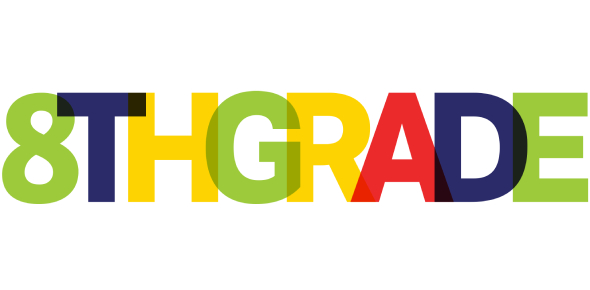# Pre Semester 2 Math Test For Grade 8(Part Two)

10 Questions | Attempts: 97
ShareSettings• 1.
11.A stone is throw up vertically from point O ,The distance from O is y meters, after x      Seconds  is given by function  y = 40x – 5x2. The maximum height of the stone is…m
• A.

A. 50

• B.

B. 60

• C.

C.75

• D.

D.80

• 2.
12.Which of the following is true for a gradient / a slope ?
• A.

A. If a straight line is parallel to the X – axis ,its gradient is zero

• B.

b. A gradient of a straight line is the ratio of horizontal distance to the vertical           distance.

• C.

A straight line has a gradient with a negative value if it inclines downwards to The left.

• D.

D.  A straight line has a gradient with a positive value if it inclines downwards to The right

• 3.
13. The parallel line to line y = 5x – 4   is …
• A.

A. y = 2x – 4

• B.

B. 5x + y = 12

• C.

C. -5x – y = 6

• D.

D. 5x – y + 3 = 0

• 4.
14.An equation of a line through points ( -1, -5 ) and has gradient of three is …
• A.

A. y = 3x + 2

• B.

B.5x + y = 12

• C.

C. -5x – y = 6

• D.

D. 5x – y + 3 = 0

• 5.
15.The gradient of a line through points of P( 1, -3 ) and Q ( 3,7) is ….
• A.

A. – 5

• B.

B. 5

• C.

C. 1/5

• D.

D. - 1/5

• 6.
16.An equation of a line through points  ( -2, 4 ) and ( 3, -2 ) is …
• A.

A. -6x + 5y -8 = 0

• B.

B. 6x + 5y -8 = 0

• C.

C. 6x + 5y – 8 = 0

• D.

D. 6x + 5y + 8 = 0

• 7.
17.Which of the following pairs of points of a straight line with a slope of  2/3 ?       I   . ( 0,-2 ) , ( 3, 2 )       II    ( 5,0 ) , ( 8,2 )       III   ( 3, 3 ) , ( -3, -1 )
• A.

A. I and II only

• B.

B. I and III only

• C.

C. II and III only

• D.

D. I , II , III

• 8.
.18. A straight line AB passes through the point ( -1, -1 ) and parallel to 4x – 2y = 5        The equation of AB is  y = ….
• A.

A. 2x – 1

• B.

B. 2x + 1

• C.

C. 4x – 1

• D.

D. 4x + 1

• 9.
19.The equation of a line that passes through the intersection point of two lines       4x + 7y – 15 = 0   and 9x – 14y – 4 = 0 perpendicular to line 2x + 5y – 3 = 0  is …
• A.

A. 2y – 5x = -1

• B.

B. 2x – 5y = -1

• C.

C. 5x – 2y = 4

• D.

D. 5x – 2y = 8

• 10.
.  20.The solution set  of  2x + y = 9  and  3x + 2y = 13    is …
• A.

A. { 5, 1 }

• B.

B. { -5, 1 }

• C.

C. { 5, -1 }

• D.

D. { -5, -1 }

## Related TopicsBack to top
×

Wait!
Here's an interesting quiz for you.